# 12.2 Planes

The (cartesian) equation of a plane is linear in the coordinates x and y, that is, of the form ax+by+cz+d=0. The normal direction to this plane is (a,b,c). The intersection of this plane with the x-axis, or x-intercept, is x=-d/a; the y-intercept is y=-d/b, and the z-intercept is z=-d/c. The plane is vertical (perpendicular to the xy-plane) if c=0; it is perpendicular to the x-axis if b=c=0; and likewise for the other coordinates.

When a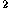+b+c=1 and d0 in the equation ax+by+cz+d=0, the equation is said to be in normal form. In this case d is the distance of the plane to the origin, and (a,b,c) are the direction cosines of the normal.

To reduce an arbitrary equation ax+by+cz+d=0 to normal form, divide by, where the sign of the radical is chosen opposite the sign of d when d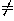0, the same as the sign of c when d=0 and c0, and the same as the sign of b otherwise.

### Planes with prescribed properties

Plane through (x,y,z) and perpendicular to the direction (a,b,c):

a(x-x)+b(y-y)+c(z-z)=0

Plane through (x,y,z) and parallel to the directions (a,b,c) and (a,b,c):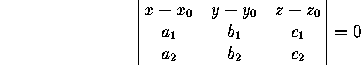Plane through (x,y,z) and (x,y,z) and parallel to the direction (a,b,c):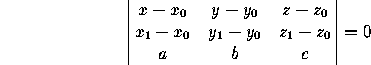Plane going through (x,y,z), (x,y,z) and (x,y,z):(The last three formulas remain true in oblique coordinates.)

The distance from the point (x,y,z) to the plane ax+by+cz+d=0 is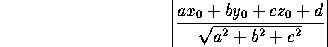The angle between two planes ax+by+cz+d=0 and ax+by+cz+d=0 is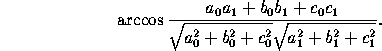In particular, the two planes are parallel when a:b:c= a:b:c, and perpendicular when aa+bb+cc=0.

### Concurrence and Coplanarity

Four planes ax+by+cz+d=0, ax+by+cz+d=0, ax+by+cz+d=0 and ax+by+cz+d=0 are concurrent if and only if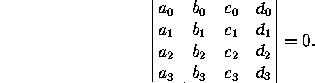Four points (x,y,z), (x,y,z), (x,y,z) and (x,y,z) are coplanar if and only if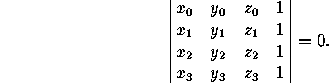(Both of these assertions remain true in oblique coordinates.)

Next: 12.3 Lines
Up: 12 DirectionsPlanes and Lines
Previous: 12.1 Direction Angles and Direction CosinesThe Geometry Center Home Page

Silvio Levy
Wed Oct 4 16:41:25 PDT 1995

This document is excerpted from the 30th Edition of the CRC Standard Mathematical Tables and Formulas (CRC Press). Unauthorized duplication is forbidden.